# UPS配套电池及UPS输入输出、电池连接线径及空开的计算方式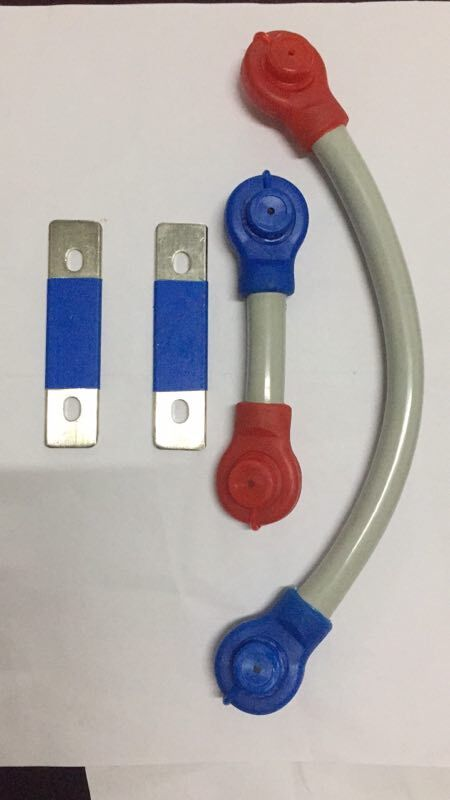PW---单机电池满足相对应时间所提供的恒功率放电

P(VA) ---UPS 容量

Pf----输入功率因数(通常采用COS0.8,

η----电池逆变器效率电池每组节数为为,要求，

*N*n-----n为并联电池组数, N为单电池组节数

主机科华60KVA ,输入功因PF=0.8,电池逆变效率η=0.92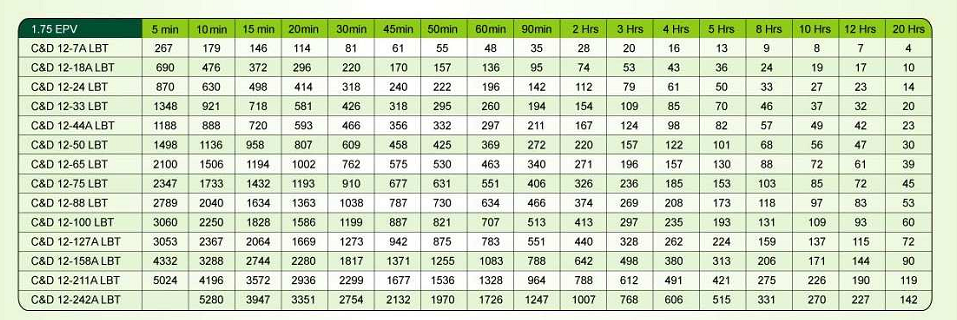P(W)=413W(理论功率)表格显示单节12V100AH电池2小时提供的恒功率放电功率

PW={PVA*Pf}/η*N*n

PW=60000VA*0.8/(0.92*4*29)=449.77W    (计算功率)

PW(计算功率)450  >P(W)=413W(理论功率)

P(w) =P(VA)*Pf/N         (计算功率)

PW---单机电池满足相对应时间所提供的恒功率放电

P(VA) ---UPS 容量

Pf----输入功率因数(通常采用COS0.8,

N---蓄电总数量

P(W)=413W(理论功率)

P(w)=P(VA)*Pf/N

P(W)=60000*0.8/116=413W

I =P(VA)*Pf/E       (计算UPS电池组最大放电电流)

E---电池组的额定电压(V)

I=60000VA*0.8COS/12V*29=137.93A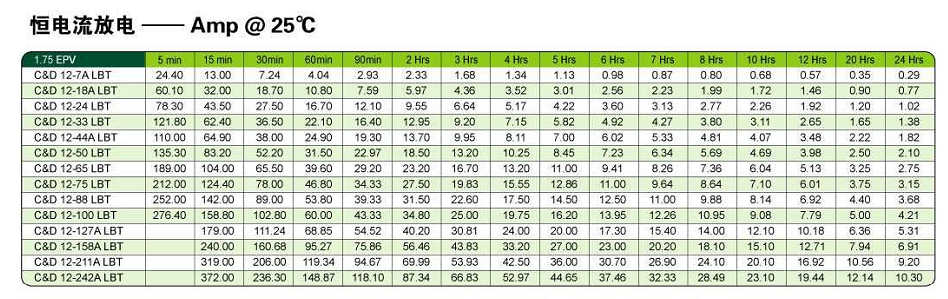12V100AH的恒电流查表所知

I(电池恒电流)=34.8A

N=I(UPS)/I(电池)=138/34.8=3.965≈4组

4*29=116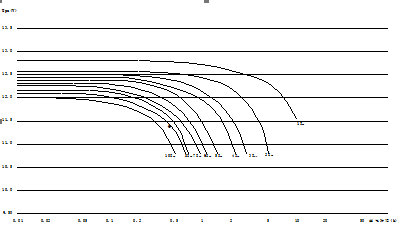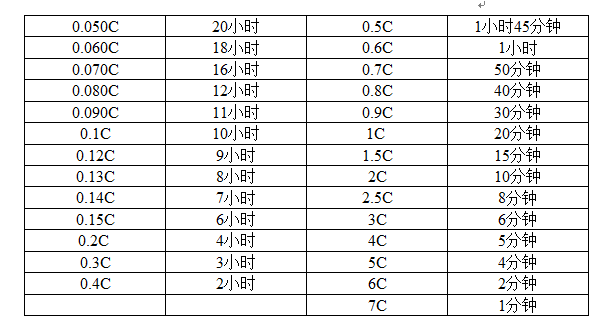IMAX=  P×cosφ      IMAX：电池组最大供电电流（A

μ×E       PUPS额定功率（VA

cosφ：负载功率因素

μ：电池逆变效率

C= IMAX              C：电池的放电速率

Q              Q：电池组的额定容量（AH

E：电池额定电

IMAX=  60000VA*0.8COS/10.5V*29*0.92=171A

C=171A/100AH*4=0.42C

1.2对应照明类负载使用；

1.5对应次级空开使用；

2.0倍对应总开使用

#### 三、电池连接线径及空开计算公式

 线径（平方毫米） 1mm² 1.5   mm 2 mm 2.5   mm 4 mm 6 mm 10   mm 可通过最大电流（安培） 10A 15A 18A 20A 28A 35A 50A 16   mm 25   mm 35   mm 50   mm 70   mm 95   mm 80A 100A 120A 160A 180A 250A 120   mm 150   mm 185   mm 200   mm 300A 350A 400A 500A

 5A 10A 16A 20A 25A 32A 63A 80A 100A 160A 180A 200A 225A 250A 300A 400A 500A 600A